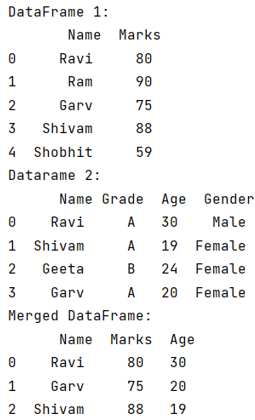# Python Pandas: Merge only certain columns

Given two Pandas DataFrames, we have to merge only certain columns.
Submitted by Pranit Sharma, on June 12, 2022

DataFrames are 2-dimensional data structures in pandas. DataFrames consist of rows, columns, and the data. DataFrame can be created with the help of python dictionaries or lists but in the real world, CSV files are imported and then converted into DataFrames. Sometimes, DataFrames are first written into CSV files. Here, we are going to merge some specific columns of DataFrames, for this purpose we are going to use pandas.DataFrame.merge() method. Also, we will pass the list of names of columns that we want to merge.

pandas.DataFrame.merge() Method

When we want to update a DataFrame with the help of another DataFrame, we use this method. This method is used to merge two DataFrames based on an index.

Syntax:

```DataFrame.merge(
right,
how='inner',
on=None,
left_on=None,
right_on=None,
left_index=False,
right_index=False,
sort=False,
suffixes=('_x', '_y'),
copy=True,
indicator=False,
validate=None
)
```

To work with pandas, we need to import pandas package first, below is the syntax:

```import pandas as pd
```

Let us understand with the help of an example how to merge some specific columns into another DataFrame.

## Python code to merge only certain columns

```# Importing pandas package
import pandas as pd

# Creating a dataframe
df1 = pd.DataFrame({
'Name':['Ravi', 'Ram', 'Garv', 'Shivam', 'Shobhit'],
'Marks':[80, 90, 75, 88, 59]}
)

# Creating another dataframe
df2 = pd.DataFrame({
'Name':['Ravi', 'Shivam', 'Geeta', 'Garv'],
'Age':[30, 19, 24, 20],
'Gender':['Male', 'Female', 'Female', 'Female']}
)

# Display df1
print("DataFrame 1:\n",df1)

# Display df2
print("Datarame 2:\n",df2)

# Merging only Name and Age column of
# df2 with df1
result = df1.merge(df2[['Name','Age']])

# Display the result
print("Merged DataFrame:\n",result)
```

Output:What's New (MCQs)

Top Interview Coding Problems/Challenges!

IncludeHelp's Blogs

Languages: » C » C++ » C++ STL » Java » Data Structure » C#.Net » Android » Kotlin » SQL
Web Technologies: » PHP » Python » JavaScript » CSS » Ajax » Node.js » Web programming/HTML
Solved programs: » C » C++ » DS » Java » C#
Aptitude que. & ans.: » C » C++ » Java » DBMS
Interview que. & ans.: » C » Embedded C » Java » SEO » HR
CS Subjects: » CS Basics » O.S. » Networks » DBMS » Embedded Systems » Cloud Computing
» Machine learning » CS Organizations » Linux » DOS
More: » Articles » Puzzles » News/Updates